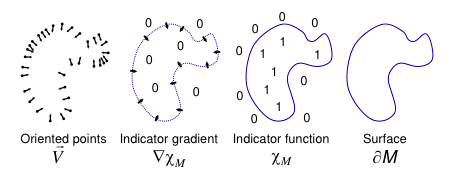## 基本思想

$\Delta \mathcal{X} = \nabla \cdot \nabla \mathcal{X} = \nabla \cdot \vec{V}$

## 具体思路

$\nabla (\mathcal{X}_M * \tilde{F})(q_0) = \intop_{\partial M} \tilde{F}_p(q_0) \vec{N}_{\partial M}(p) dp$

（原文中有证明）

\begin{aligned} \nabla (\mathcal{X}_m * \tilde{F})(q) &= \sum_{s \in S} \intop_{\mathscr{P}_s} \tilde{F}_p(q) \vec{N}_{\partial M}(p)dp \\ &\approx \sum_{s \in S} | \mathscr{P}_s | \tilde{F}_{s.p}(q) s.\vec{N} \equiv \vec{V}(q) \end{aligned}

1. 足够窄，不至于过平滑（over-smooth）
2. 足够宽，可以很好的通过由块面积尺度化的在$s.p$处的值估计$\mathscr{P}_s$上的积分

$\Delta \tilde{\mathcal{X}} = \nabla \cdot \vec{V}$

## 实现

### 离散化

1. 向量场$\vec{V}$可以用$F_o$的线性和精准有效地表示
2. $F_o$项表示的泊松方程矩阵形式可以被有效求解
3. 指示函数作为$F_o$和的形式可以在模型曲面附近被精准有效地估计

#### 定义函数空间

$F_o(q) \equiv F \left( \frac{q - o.c}{o.w} \right) \frac{1}{o.w^3}$

#### 选择基函数

$F(q) = \tilde{F} \left( \frac{q}{2^D} \right)$

1. 得到的散度和拉普拉斯算子是稀疏的
2. 估计在点$q$处表示为$F_o$线性和的函数，仅仅需要将在$q$比较近的节点$o \in \mathscr{O}$上求和。

$F(x,y,z) \equiv (B(x)B(y)B(z))^{*n} ~~ \text{with} ~~ B(t) = \begin{cases} 1 & |t| < 0.5 \\ 0 & \text{otherwise} \end{cases}$

$n$增长的时候，$F$更接近高斯并且支持范围更大；在该文的实际实现中使用$n=3$

### 向量场定义

$\vec{V}(q) \equiv \sum_{s \in S} \sum_{o \in \text{Ngbr}_D(s)} \alpha_{o,s} F_o(q) s.\vec{N}$

### 泊松求解

$\sum_{o \in \mathscr{O}} \| \langle \Delta \tilde{\mathcal{X}} - \nabla \cdot \vec{V}, F_o \rangle \|^2 = \sum_{o \in \mathscr{O}} \| \langle \Delta \tilde{\mathcal{X}}, F_o \rangle - \langle \nabla \cdot \vec{V}, F_o \rangle \|^2$

$L_{o,o'} \equiv \langle \frac{\partial^2 F_o}{\partial x^2}, F_{o'} \rangle + \langle \frac{\partial^2 F_o}{\partial y^2}, F_{o'} \rangle + \langle \frac{\partial^2 F_o}{\partial z^2}, F_{o'} \rangle$

$\min_{x \in \mathbb{R}^{|\mathscr{O}|}} \| Lx - v \|^2$

### 等值面提取

$\partial \tilde{M} \equiv \{ q \in \mathbb{R}^3 | \tilde{\mathcal{X}}(q) = \gamma \} ~~ \text{with} ~~ \gamma = \frac{1}{|S|} \sum_{s \in S} \tilde{\mathcal{X}} (s.p)$

### 非均匀采样

#### 估计局部采样密度

$W_{\hat{D}}(q) \equiv \sum_{s \in S} \sum_{o \in \text{Ngbr}_{\hat{D}}(s)} \alpha_{o,s}F_o(q)$

#### 计算向量场

$\vec{V}(q) \equiv \sum_{s \in S} \frac{1}{W_{\hat{D}} (s.p)} \sum_{o \in \text{Ngbr}_D(s)} \alpha_{o,s} F_o(q) s.\vec{N}$

$\vec{V}(q) \equiv \sum_{s \in S} \frac{1}{W_{\hat{D}} (s.p)} \sum_{o \in \text{Ngbr}_{\text{Depth}(s.p)}(s)} \alpha_{o,s} F_o(q) s.\vec{N}$

$\text{Depth}(s.p) \equiv \min (D, D + \log_4(W_{\hat{D}} (s.p) / W))$

#### 选取提取等值面的值

$\tilde{\mathcal{X}}$在采样点处的加权平均值：

$\partial \tilde{M} \equiv \{ q \in \mathbb{R}^3 | \tilde{\mathcal{X}}(q) = \gamma \} ~~ \text{with} ~~ \gamma = \frac{\sum \frac{1}{W_{\hat{D}}(s.p)} \tilde{\mathcal{X}}(s.p)}{\sum \frac{1}{W_{\hat{D}}(s.p)}}$